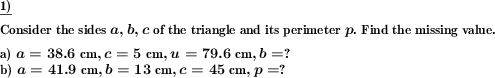Custom math worksheets at your fingertipsDetails for problem "Triangle sides and perimeter"

Quickname: 7677

Junior High School, Middle School, High School.

Summary

For a given triangle, the sides and/or perimeter have to be determined.

ExampleDescription

Use this problem in your worksheets to calculate the perimeter or any of the missing side in a triangle when all other values are given. For a triangle, the perimeter is found by adding the lengths of the three sides. In this problem set, one of these values (side a, side b, side c, perimeter) is missing, the other three are given. The missing value has to be calculated from the other ones. This kind of worksheet can be used as a small or whole group activity or independent/ homework practice for mastery of finding the perimeter of a triangle using sides. Practice this problem in variation to boost the objectives given below.

1. Recall the addition of decimal numbers.
2. The formula to find the perimeter of a triangle is revised.
3. Understand the use of measurement units.
4. Buildup concept of deriving required value from a given equation.
5. Develop algebraic skills in geometry.

The complexity level can be personalized to use these worksheets from grade 7 and grade 8.

• The number of problems is selectable from 1 to 10.
• The values to be asked for can be chosen:
• One of the sides only, sides or perimeter, or only the perimeter.

The answer key will include the solution of missing value.

Download free printable worksheets for this math problem here. The worksheet contains the problems only, the solution sheet includes the answers. Just click on the respective link.

•Worksheet 1Solution sheet with answers
•Worksheet 2Solution sheet with answers
•Worksheet 3Solution sheet with answers

If you can not see the solution sheets for download, they may be filtered out by an ad blocker that you may have installed. If this is the case, please allow ads for this page and reload the page. The solution sheets will then reappear.

• Do these sample worksheets do not really fit?
• Do you need more math worksheets, with a different level of difficulty?
• Would you like to combine different problems on a worksheet and adjust them to your needs?
• As a teacher, you can put together your own worksheets using the automatically generated math problems provided.
With a free initial credit, you can start creating your own math worksheets in a few minutes.

You can try it for free! Register here, to create custom worksheets now!

Customization options for this problem

Parameter
Possible values
Number of problems
1, 2, 3, 4, 5, 6, 7, 8, 9, 10
sides, perimeter, sides, perimeterDeutsche Version dieser Aufgabe
These informational pages with samples describe math problems that can be combined on custom math worksheets with solutions for home and K-12 school use.
Deutsche Seiten
×